### Home > CALC > Chapter 3 > Lesson 3.1.2 > Problem3-34

3-34.
1. In many textbooks the derivative (or IROC) is described in terms of x and ∆x (delta x) instead of x and h. Homework Help ✎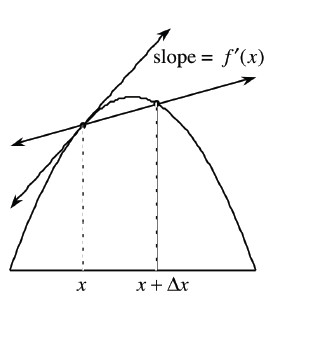1. Explain why h and ∆x are equivalent.

2. Use the picture above and the definition of IROC to write the slope of the tangent line in terms of x and ∆x.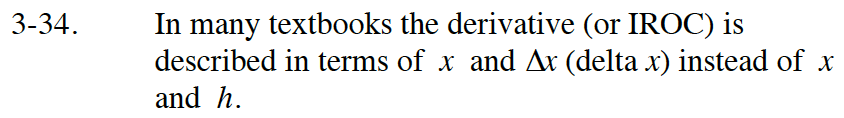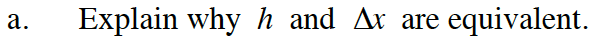Δx can be translated as 'change in x.' h typically appears in the expression: (x + h) −x. How are these equivalent?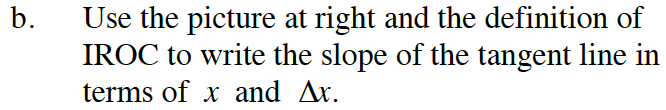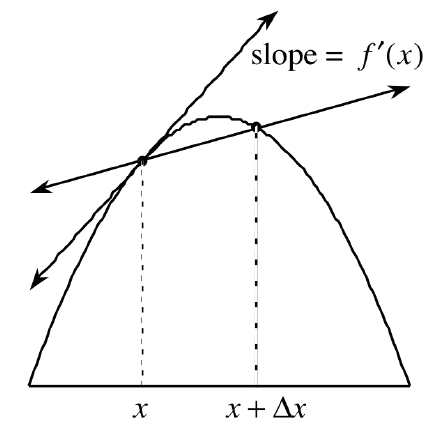'Definition of the Derivative' is the same thing as 'Instantaneous Rate of Change': IROC

$f'(x)=\lim_{h\rightarrow 0}\frac{f(x+h)-f(x)}{(x+h)-h}=\lim_{h\rightarrow 0}\frac{f(x+h)-f(x)}{h}...$